• +91 9971497814
• info@interviewmaterial.com

# RD Chapter 1- Real Numbers Ex-1.3 Interview Questions Answers

### Related Subjects

Question 1 :
Express each of the following integers as a product of its prime factors :
(i) 420
(ii) 468
(iii) 945
(iv) 7325

(i) 420
=2 x 2 x 3 x 5 x 7
= 22 x 3 x 5 x 7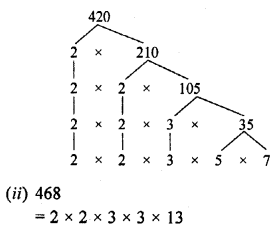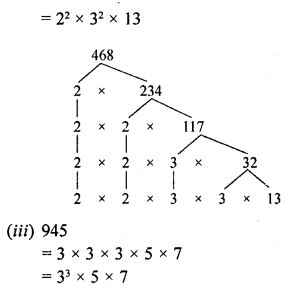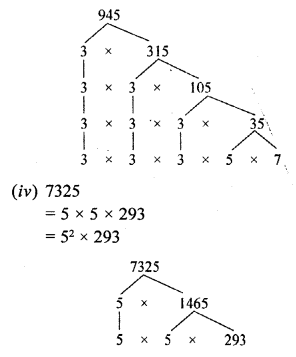Question 2 :
Determine the prime factorization of each of the following positive integer :
(i) 20570
(ii) 58500
(iii) 45470971

(i) 20570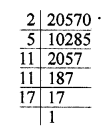20570 = 2 x 5 x 11 x 11 x 17 = 2 x 5 x 112 x 17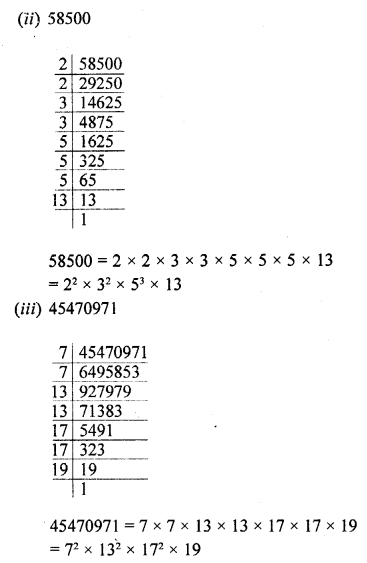Question 3 : Explain why 7 x 11 x 13 + 13 and 7 x 6 x 5 x 4 x 3 x 2 x 1 + 5 are composite numbers ?

We know that a composite number is that number which can be factorize. It has more factors other than itself and one
Now, 7 x 11 x 13 + 13 = 13 (7 x 11 + 1) = 13 x 78
Which is composite number
Similarly,
7 x 6 x 5 x 4 x 3 x 2 x 1 + 5 = 5(7 x 6 x 4 x 3 x 2 x 1 + 1)
= 5 x 1009
Which is a composite number
Hence proved

Question 4 : Check whether 6n can end with the digit 0 for any natural number n.

No, 6n can’t endwith the digit 0 as the number ending 0 can be factorise of the type
2n x 5m onlybut 6n = (2 x 3)n = 2n x3n
Which does not has 5m as factors.

Question 5 : Explain why 3 x 5 x 7 + 7 is a composite number. [NCERT Exemplar]

We have, 3 x 5 x 7 + 7 = 105 + 7 = 112
Now, 112 = 2 x 2 x 2 x 2 x 7 = 24 x7
So, it is the product of prime factors 2 and 7. i.e., it has more than twofactors.
Hence, it is a composite number.

Todays Deals### RD Chapter 1- Real Numbers Ex-1.3 Contributorskrishan

Name:
Email:

# Latest News# 9000 interview questions in different categories